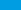# 5 Tips on How to Score Higher on SAT MathDoes your heart skip a beat every time you come across an algebraic formula in a SAT Math test? Does your mind go offshore when someone asks you the area of a pentagon? Do you shudder with the sound of SAT Math exams? According to SAT experts, you don’t need to worry about the SAT Math section! There are several ways of approaching the tacky SAT Math problems. In this short write up, you will read about five such strategies that will help you handle any SAT Math section easily.

## Tip #1 – Know What You Know

For more than several decades, you would have being asked to answer almost every problem in the question paper. This clearly proves the fact that you should make wild guesses and dark picks. However, the foremost theory doesn’t work well with SAT Math. You should not make dark guesses in the SAT Math Section. Instead, divide and conquer! That is because you are likely to lose points for every wrong answer you select. To avoid negative scores, you must fine-tune your energy and answer what you are confident about. It would be wise to answer easy questions and then move onto difficult ones.

## Tip #2 – Look Around

Secondly, you should understand the fact that the answer you want is right in front of you! The correct answer is not hidden or meant to be found. Instead of breaking your head with big equations and complicated calculations, you should learn to pick the right answer. Get rid of answers that won’t fit with the problem. This is an enticing move that can save you both time and effort.

## Tip #3 – Going Backwards

When it comes to complicated word problems, you should stick onto the answer first technique. This is a powerful SAT Math strategy that revolves around working backwards. If you manage to find the right answer in your first 2 guesses, CONGRATS! If not, you are just few steps away from the correct pick.

## Tip #4 – Let’s Estimate

What is America’s much loved pastime? What you are about to deal with would be “Estimating”. This is a useful tool in many SAT Math problems. A quick fact is to draw diagrams and find solutions to the problem. A simple sketch can tell you many things.

## Tip #5 – Understanding Math

Last but certainly not least, you should understand the Math language and algebra. There is a thin line between Math and normal English. Well, if you are nostalgic about MLA, you will have a tough time-solving SAT Math problems. This is why you should become familiar and learn how to decipher the math language.

Looking for a great resource to help you or your student ace the PSAT Math test? This product is a perfect bundle for everything a PSAT Math test taker will ever need to ace the PSAT Math test.

### PSAT Math Comprehensive Prep Bundle: A Perfect Resource for PSAT Math Test Takers